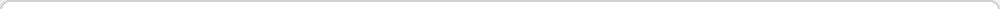# Annually Compounded Dividend Calculator

Dividend Reinvestment is where you reinvest your dividends in the same stock that issues the dividend originally, then the next time the dividend is issued you have more shares, so your dividend is higher, and you reinvest more, thus gaining more shares. This is called compounding, and can make you very wealthy in the long term. The more frequent the distributions, the more frequent the compounding, the more money you will make. This calculator is an annually compounded dividend calculator it is the "slowest" calculator we have showing you what you can earn with a stock that just issues their dividend once per year. Run your numbers with it then consider comparing to our Quarterly Compounded Dividend Calculator or our Monthly Compounded Dividend Calculator to see how the compounding schedules result in varying amounts of income.

 Starting Yield: % (the current annual dividend yield, such as 4.5) Dividend Growth Rate: % (the historical average increase in the dividend as a percentage, you may set this to 0 to disregard it) Number of Shares Held: (the number of shares you own in the stock) Cost per Share: \$ (your purchase price for the shares) Years to hold: (the number of years for which you want to calculate results)